#### How to test Statistical Significance by T-test?

A t-test is a statistical test that helps to determine if there is a significant difference between the means of two sets of data. In MS Excel, we can easily test statistical significance by using the function TTEST. Let’s first understand the parameters of this function.

## Ttest function syntax

=TTEST(array1, array2, tails, type)

Array1 (Required): Range for dataset 1

Array2 (Required): Range for dataset 2

Tails (Required): This determines if it is a one-tail T-test or two tail T-test.

• 1: One tail T-test
• 2: Two tail T test

Type (required): Type of t-test

• 1: Paired T-test, i.e. if the two data sets are related to each other
• 2: Unpaired T-test, the two samples have equal variance
• 3: Unpaired T-test , the two samples have unequal variance

## Ttest formula example

Example:

1. Consider the following dataset for T-test.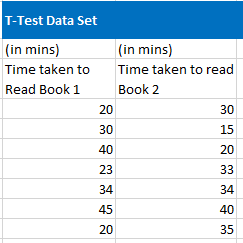2. Select the two –datasets in array 1 and array 2.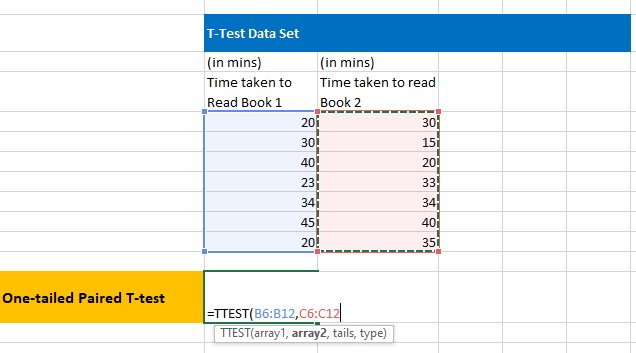3. On an Empty Cell, write the formula to perform 1-tail Paired T test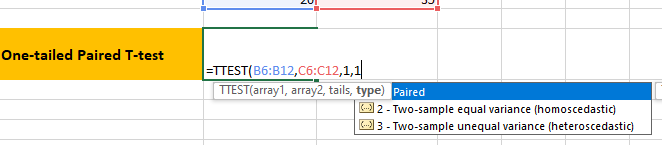One-tailed probability obtained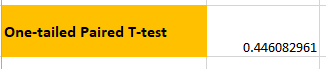4. Similarly, perform a two–tailed paired T test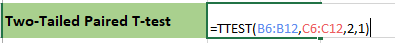Two-tailed probability obtained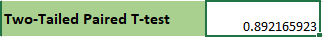Based on the obtained value you can accept or reject the null hypothesis. If the T-test gives the probability less than 0.05, then reject the null hypothesis. This means there is less than 5% probability that the null hypothesis is true.

Here, in both cases, p-value> 0.05. So, we accept the null hypothesis.

## Performing a t-test using the “Data Analysis”

In Microsoft Excel, you can also perform a t-test using the “Data Analysis” tool. Here are the steps to perform such a t-test in Excel:

1. Prepare your data: Organize your data into two columns, one for each group that you want to compare. Make sure that the data is clean and free of errors.
2. Open the Data Analysis Tool: Click on the “Data” tab in the ribbon menu and then click on “Data Analysis” in the “Analysis” section. If the “Data Analysis” tool is not visible, you may need to install it. To do so, go to “File” > “Options” > “Add-ins” > “Excel Add-ins” and then select “Analysis ToolPack”.
3. Select the “t-Test: Two-Sample Assuming Equal Variances” option: In the “Data Analysis” dialog box, select “t-Test: Two-Sample Assuming Equal Variances” and then click on the “OK” button.
4. Enter the input range: In the “t-Test: Two-Sample Assuming Equal Variances” dialog box, enter the input range for the two columns of data. Make sure to select the correct range, including the labels if they exist.
5. Select the output options: In the same dialog box, select the output options that you want, such as the location of the output, whether to display descriptive statistics, and whether to display the pooled variance.
6. Click on the “OK” button: Excel will now perform the t-test and display the results.